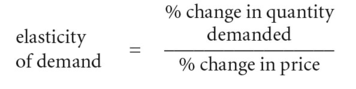# price-elasticity of demand

## price-elasticity of demand

a measure of the degree of responsiveness of DEMAND to a given change in PRICE:If a change in price results in a more than proportionate change in quantity demanded, then demand is price-elastic (Fig. 148 (a)); if a change in price produces a less than proportionate change in the quantity demanded, then demand is price-inelastic (Fig. 148).

At the extremes, demand can be perfectly price-inelastic, that is, price changes have no effect at all on quantity demanded, which shows up as a straight-line vertical demand curve; or demand can be perfectly price-elastic, that is, any amount will be demanded at the prevailing price, which shows up as a straight-line horizontal demand curve.

TOTAL REVENUE (price times quantity demanded) will be affected by price changes. Where demand is price-elastic, a small cut in price will generate a large increase in quantity demanded so the price cut will serve to increase total revenue (while a price rise serves to reduce total revenue). By contrast, where demand is price-inelastic, a large cut in price will generate only a small increase in quantity demanded so the price cut will serve to reduce total revenue (while a price rise serves to increase total revenue). Where demand has unitary elasticity, then the percentage price cut will be matched by an exactly offsetting percentage change in quantity demanded so that total revenue remains the same.

It must be stressed, however, that the price-elasticity of demand can vary along the length of a given demand curve. For example, although in Fig. 148 (a) the demand curve as a whole is elastic in configuration, it is more elastic at point X than it is at point Y.

The concept of demand elasticity is useful to firms in setting product price (including PRICE DISCRIMINATION) and to governments in setting rates of indirect taxes, such as EXCISE DUTY, to raise revenue. See also ARC ELASTICITY, POINT ELASTICITY, INCIDENCE OF TAXATION, TARIFF, PENETRATION PRICE, SKIMMING PRICE, MARSHALL-LERNER CONDITION.

Collins Dictionary of Economics, 4th ed. © C. Pass, B. Lowes, L. Davies 2005
References in periodicals archive ?
Israel points out that "price-elasticity of demand" is essential in understanding how prices (and costs) are formed.
We define [[member of].sup.o] [greater than or equal to] 0 and [[member of].sup.a] [greater than or equal to] 0 as the absolute value of the price-elasticity of demand for fuel products for country o and country a, respectively.
Not surprisingly, a reduction in [P.sub.s] would lead to an increase in consumption of oil products in country o, with the size of the response being determined by its price-elasticity of demand.
Under a CES aggregator, the final goods bundle is given by Y = [([[integral].sup.N.sub.0] [y.sup.([theta] - 1)/[theta].sub.j] dj).sup.[theta]/([theta] - 1)], where [theta] > 1 is the constant price-elasticity of demand in absolute value.
Under a translog preference structure as in Feenstra (2003), the price-elasticity of demand is increasing in the number of differentiated goods, 1 + [gamma]N, where [gamma] > 0 measures the price-elasticity of the spending share on an individual good and both price-elasticities are expressed in absolute value.
Even if negotiated prices were observed, these prices do not directly determine the price-elasticity of demand, which instead depends upon the amount paid by the relevant decisionmaker, the patient.
(66) In the logit model the price-elasticity of demand that a particular hospital j faces in a specific submarket is [e.sub.j] = k f([s.sub.i], ..., [s.sub.j], ...
But they left tolls for heavy vehicles almost unchanged relative to others, since more damage called for higher prices but higher price-elasticity of demand justified lower prices.
"Regional differences in the price-elasticity of demand for energy." subcontract report, NREL/SR-620-39512, RAND Corporation Santa Monica, California, http://www.nrel.gov/docs/fy06osti/39512.pdf, (download 10-15-2011)
"Regional Differences in the Price-Elasticity of Demand for Energy", Subcontract Report, NREL/SR-620-39512, RAND Corporation Santa Monica, California, http://www.nrel.gov/docs/fy06osti/39512.pdf, (download 10-15-2011).

Site: Follow: Share:
Open / Close